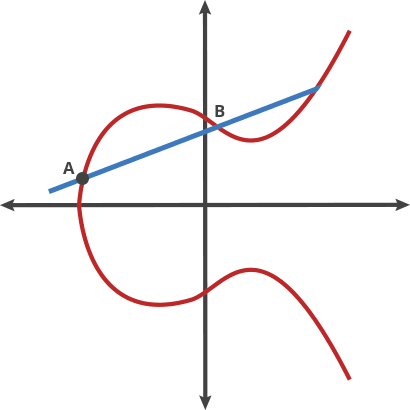Search

# Elliptic-curve Cryptography (ECC)

Last updated Jun 23, 2022 Edit Source

• A form of asymmetric cryptography
• Much smaller key-sizes than RSA
• Elliptic Curve properties (specifically looking at Ed25519)
• Formula follows something like $y^2 = x^3 + ax + b$
• Symmetric about the x-axis
• Drawing a straight line through the curve will intersect no more than 3 points
• A line between any two points will also intersect the curve at another place
• Starting point on the curve point: A
• “dot” function -> kind of like a game of billiards
• In this game of billiards, you take a ball at point A, shoot it towards point B. When it hits the curve, the ball bounces either straight up (if it’s below the x-axis) or straight down (if it’s above the x-axis) to the other side of the curve. The point it lands on is C
• If the value C is over some maximum value (usually a prime), we modulus it with the maximum to end with a valid number.
• A dot B = C
•• It turns out that if you “dot” an initial point with itself $n$ times to arrive at a final point, finding out $n$ when you only know the final point and the first point is hard
• $n$ is then the private key, point A dotted with itself $n$ times is the public key
• ECDHE stands for Elliptic Curve Diffie Hellman Ephemeral and is a key exchange mechanism based on elliptic curves
• Curve25519 is a popular set of elliptic curve parameters and reference implementation by Daniel J. Bernstein in C. Bindings and alternative implementations are also available.